Fingerings Home Octave 1: F  (C) Gb  (Db) G  (D) Ab  (Eb) A  (E) Bb  (F) B  (Gb) C  (G) Db  (Ab) D  (A) Eb  (Bb) E  (B) Octave 2+: F  (C) Gb  (Db) G  (D) Ab  (Eb) A  (E) Bb  (F) B  (Gb) C  (G) Db  (Ab) D  (A) Eb  (Bb) E  (B) F  (C) Gb  (Db) G  (D)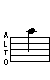A (E) - 2nd Octave## Trills

Trill - From B   Trill - From Bb
B A   Bb A (alt)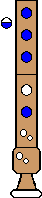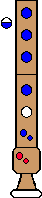Top

## Turned Trills

Turned Trill
Full step above, Full step below
B A G (alt) A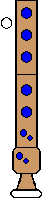Top

Turned Trill
Half step above; Full step below
Bb A (alt) G (alt) ATop

Turned Trill
Full step above; Half step below
B A Ab A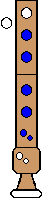Top

Turned Trill
Half step above; Half step below

(See below for alternate)
Bb A (alt) Ab AComments: Kind of difficult - awkward.
Alternate
Turned Trill
Half step above; Half step below
Bb A Ab AComments: This uses a B-to-A ("wide") trill to make it somewhat easier. Is this cheating?
Top

## Regular Turns

Turn
Full step above; Full step below
B A G (alt) ATop

Turn
Half step above; Full step below
Bb A G (alt) ATop

Turn
Full step above; Half step below
B A Ab A